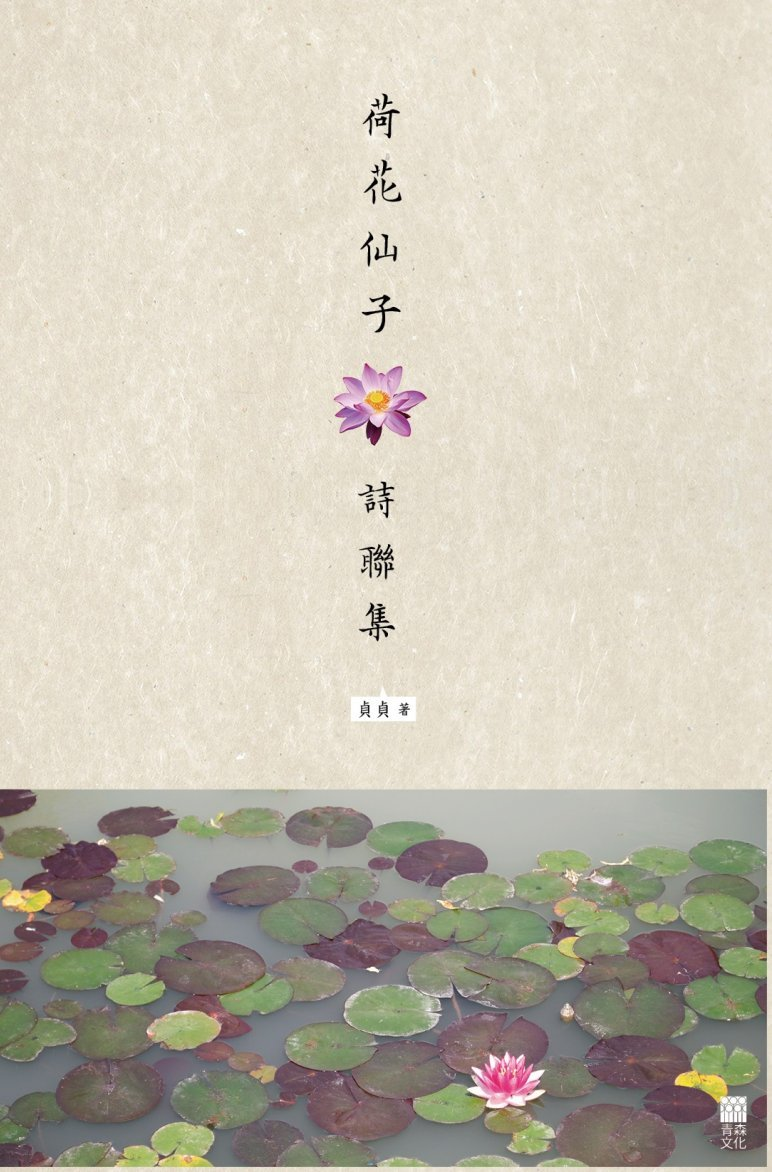# 荷花仙子詩聯集

• 紙書

### 本書特色

• 內容包含五言古體詩、五言絕句、五言律詩、七言古體詩、七言絕句、七言律詩、排律（長律）、近體詩（格律詩）、四言和五言對聯、六言和七言對聯，以及長對聯，是一本不錯的入門級詩聯集。
• 特別收錄作者甚為欣賞的古今奇聯及千古絕對與讀者分享。
• 個人版稅收入將全數撥作慈善用途，用以資助中國內地山區兒童之學習及教育等。

### 貞貞

• 目錄
• 前言
• 詩聯簡介
• 卷一 五言古體詩
• （一）報曉
• （二）白天鵝
• （三）翱翔天際
• （四）無言
• （五）雪月迷情
• （六）送暖
• 卷二 五言絕句
• （七）詠荷（一）
• （八）詠荷（二）
• （九）歲月神偷
• （十）無花果
• （十一）蝶夢遙
• （十二）黃沙情
• （十三）畫苑新花
• （十四）飛鴻踏雪泥
• （十五）彼岸花
• （十六）火龍果
• （十七）明心
• （十八）平湖秋月
• （十九）衍聯詩─夏夢梵音
• （二十）衍聯詩─竹荷
• （二十一）衍聯詩─雁影殘秋
• 卷三 五言律詩
• （二十二）重陽節
• （二十三）嵌名詩─蕙嫦高雅朝暉才女
• （二十四）恩師頌
• 卷四 七言古體詩
• （二十五）桃花仙子
• （二十六）薰衣草
• （二十七）流星
• （二十八）採花蝶兒
• （二十九）盼郎歸
• （三十）石榴
• （三十一）竝蒂蓮
• （三十二）孔雀
• （三十三）莫負青春
• （三十四）水鄉春色
• （三十五）鶯報春來
• （三十六）小白鵝
• 卷五 七言絕句
• （三十七）本句回文詩─秋聲
• （三十八）通體回文詩─思鄉（順讀）
• （三十九）通體回文詩─思鄉（倒讀）
• （四十）十字詩*疊字回文詩─幽泉月鼓
• （四十一）十字詩*疊字回文詩─曉岸啼鶯
• （四十二）十字詩*疊字回文詩─九疊雲屏
• （四十三）十字詩*疊字回文詩─落桂飄香
• （四十四）十字詩*疊字回文詩─滴翠凝珠
• （四十五）十字詩*疊字回文詩─映日輕煙
• （四十六）十字詩*疊字回文詩─泣丹楓
• （四十七）十字詩*疊字回文詩─疊翠流金
• （四十八）十字詩*疊字回文詩─斷藕絲連
• （四十九）連環詩─岫雲遊
• （五十）連環詩─搖香暗繞
• （五十一）數字詩─百鳥林
• （五十二）數字詩─心曲
• （五十三）衍聯詩─春去秋又來
• （五十四）衍聯詩─紅塵一轉
• （五十五）衍聯詩─雪影迷情
• （五十六）奇聯妙畫
• （五十七）秋霜
• （五十八）秋波
• （五十九）含羞草
• （六十）春臨
• （六十一）秋月離雁
• （六十二）鸚鵡洲秋色
• （六十三）弄潮人
• （六十四）春滿人間
• （六十五）晚暉
• （六十六）醉會同窗
• （六十七）燕歸來
• （六十八）康河廝守
• （六十九）平湖秋月
• （七十）柳浪聞鶯
• （七十一）我為卿狂
• （七十二）我愛楓狂
• （七十三）元宵佳節
• （七十四）元宵情人節
• （七十五）簫聲
• （七十六）日映蓮薌
• （七十七）雪中緣
• （七十八）詠竹
• （七十九）上善若水
• （八十）寂夜愁思
• （八十一）夕陽美景
• （八十二）癡情恨
• （八十三）文章何價
• （八十四）深閨夢裏人
• （八十五）春泥
• （八十六）春蠶破繭
• （八十七）隨筆
• （八十八）鯉躍龍門
• （八十九）學勤
• （九十）白雲
• 卷六 七言律詩
• （九十一）端午節
• （九十二）中秋月
• （九十三）秋思（一）
• （九十四）秋思（二）
• （九十五）緣來緣去
• （九十六）清暉園遊記
• （九十七）緣
• （九十八）赤壁吟
• （九十九）諸葛武鄉侯
• （壹百）諸葛武鄉侯（古體詩改寫成律詩）
• （壹百零一）清江夏雨曲
• （壹百零二）情寄桃花源
• （壹百零三）斷掌紋人
• （壹百零四）中秋夜
• 卷七 排律（長律）
• （壹百零五）醜小鴨
• *乾隆下江南*二十六字詩*─清*乾隆
• 卷八 近體詩（格律詩）平仄譜式
• 卷九 四言、五言對聯
• 卷十 六言、七言對聯
• 卷十一 長對聯
• 卷十二 古今奇聯欣賞
• 卷十三 千古絕對欣賞
• 作者介紹